MacKenzie, I. S., & Buxton, W. (1992). Extending Fitts' law to two-dimensional tasks. Proceedings of the CHI'92 Conference on Human Factors in Computing Systems, pp. 219-226. New York: ACM.

# Extending Fitts' Law to Two-Dimensional Tasks

## I. Scott MacKenzie1 and William Buxton2

1Department of Computing and Information Science
University of Guelph
519-824-4120, mac@snowhite.cis.uoguelph.ca

2Dynamic Graphics Project, Computer Systems Research Institute
University of Toronto
416-978-1961, willy@dgp.toronto.edu

## ABSTRACT

Fitts' law, a one-dimensional model of human movement, is commonly applied to two-dimensional target acquisition tasks on interactive computing systems. For rectangular targets, such as words, it is demonstrated that the model can break down and yield unrealistically low (even negative!) ratings for a task's index of difficulty (ID). The Shannon formulation is shown to partially correct this problem, since ID is always >= 0 bits. As well, two alternative interpretations of "target width" are introduced that accommodate the two-dimensional nature of tasks. Results of an experiment are presented that show a significant improvement in the model's performance using the suggested changes.

KEYWORDS: human performance modeling, Fitts' law, input devices, input tasks.

## INTRODUCTION

Since the advent of direct manipulation human-computer interfaces (using, for example, the mouse), research in human performance on computing systems has enlisted many traditional techniques and models from human factors. An example is Fitts' law, a speed-accuracy model of human movement developed from research in man-machine systems for air traffic control [4, 5].

In early applications of the law, an operator manipulated a control (e.g., a lever, slider, or rotary knob) over a specified amplitude to a terminal position of a specified accuracy [e.g., 13]. Since the 1970s, many researchers have adopted a different paradigm. Objects of interest are often "iconic" -- represented on a two-dimensional CRT display and selected by a mouse, joystick, or trackball [e.g., 2, 3, 12]. Unfortunately, and as we shall demonstrate, the law is inherently one-dimensional; so, many such experiments include confounding variables such as approach angle and target shape.

The contribution of the present paper is in extending Fitts' law to 2D target acquisition tasks and in alleviating common weaknesses in applying the model. Following a brief introduction, we demonstrate -- with examples from published research -- that inaccurate, even erroneous, measures often emerge in typical applications of the model. Modifications are introduced and an experiment is presented to compare alternative models.

## FITTS' LAW

According to Fitts' law, the time (MT) to move to and select a target of width W which lies at distance (or amplitude) A is
`     MT = a + b log2(2A/W)     (1)`
where a and b are constants determined through linear regression. W corresponds to "accuracy" -- the required region where an action terminates. The log term is the index of difficulty (ID) and carries the unit "bits" (because the base is 2). If MT is measured in "seconds", then the unit for a is "seconds" and for b, "seconds/bit". The reciprocal of b is the index of performance (IP) in "bits/second". This is the human rate of information processing for the movement task under investigation.

Variations of the law have been proposed by Welford ,

MT = a + b log2(A/W + 0.5),     (2)

and MacKenzie [10, 11],

`     MT = a + b log2(A/W + 1).     (3)`
These equations differ only in the formulations for ID. On the whole, Equation 3, known as the Shannon formulation, is preferred because it
• provides a slightly better fit with observations,
• exactly mimics the information theorem underlying Fitts' law, and
• always gives a positive rating for the index of task difficulty.

### The Geometry of Input Tasks

The experiments conducted by Fitts tested human performance in making horizontal moves toward a target. As seen in Figure 1, both the amplitude of the move and the width of the terminating region are measured along the same axis. It follows that the model is inherently one-dimensional. This implies that the minimum A is W/2, otherwise the starting position is inside the target.Figure 1. Fitts' law paradigm. The law is inherently one-dimensional
since target amplitude (A) and width (W) are measured along the same axis.

Most Fitts' law research employs a task paradigm consistent with Figure 1 [e.g., 9, 14, 12]. However, many investigations vary the angle of approach to "smooth over" or to investigate directional effects [e.g., 1, 3, 8]. If the targets are circles (or perhaps squares), then the 1D constraint in the model remains largely intact (because the "width" of a circle is the same, regardless of the angle of measurement). However, if targets are rectangles, such as words, the situation is confounded. The amplitude is still the distance to the centre of the target; but the role of target width is unclear. This is illustrated in Figure 2.

If the approach angle is 0° (Figure 2a), then the 1D scenario applies. If the approach angle is 90° (Figure 2b), then the roles of width and height reverse (from the perspective of the model). Unfortunately, this has not been accommodated in past research. Card et al. , for example, tested several devices in a text selection task and varied the angle of approach. The horizontal measurement was always considered the target "width", regardless of approach angle; so, some unusual interpretations of task difficulty (ID) emerged. For example, when selecting a 10-character (2.46 cm) target from a distance of 1 cm, ID was calculated using Welford's formulation as log2(A/W + 0.5) = log2(1/2.46 + 0.5) = -0.14 bits. This unreasonable value, although not explicitly cited, appeared in the scatter plot of MT vs. ID (Fig. 6, p. 609). In another Fitts' law experiment using similar conditions, Gillan, Holden, Adam, Rudisill, and Magee  required subjects to select a target 26 characters (6 cm) wide from a distance of 2 cm. Again, Welford's formulation was used, so ID was log2(2/6 + 0.5) = -0.26 bits.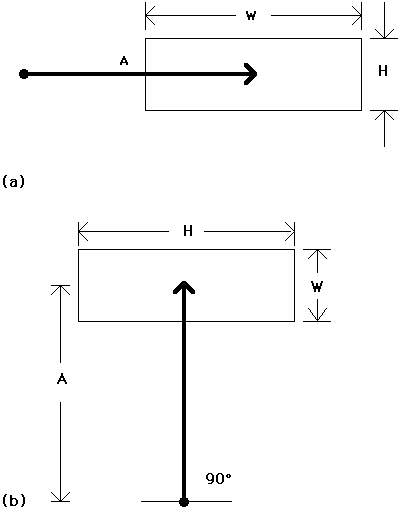Figure 2. Fitts' law in 2D. The roles of width and height reverse as
the approach angle changes from 0°. to 90°.

Obviously, a negative rating for task difficulty poses a serious theoretical problem. (What does "negative difficulty" mean?). We suggest two complementary ways to correct this. The first is to use the Shannon formulation in calculating ID (see Equation 3). For example, under the condition cited above (A = 2 cm, W = 6 cm), ID becomes log2(2/6 + 1) = +0.42 bits. It is easily shown that the Shannon formulation always yields a positive (or zero) ID. Using the Fitts or Welford formulation, however, the rating is negative when the A:W ratio drops below 1:2.

A second and additional strategy is to substitute for W a measure more consistent with the 2D nature of the task. Consider Figure 3. The inherent 1D constraint in the model is maintained by measuring W along the approach axis. This is shown as W' (read "W prime") in the figure. Notwithstanding the assertion that subjects may "cut corners" to minimize distances, the W' model is appealing because it allows a 1D interpretation of a 2D task.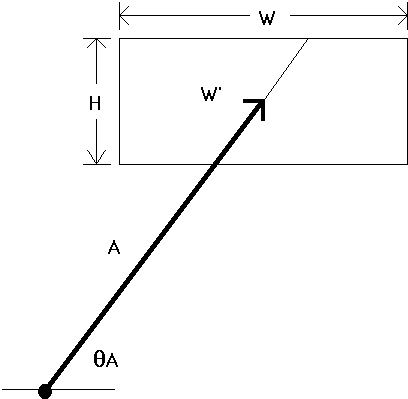Figure 3. What is target width? Possibilities include W' (the
width of the target along an approach vector) or the smaller of W or H.

Another possible substitution for target width is "the smaller of W or H". This pragmatic approach has intuitive appeal in that the smaller of the two dimensions seems more indicative of the accuracy demands of the task. We call this the "SMALLER-OF" model. This model is computationally simple since it can be applied only knowing A, W, and H. The W' model, on the other hand, requires A, W, H, THETA-A, and a geometric calculation to determine the correct substitution for W (see Figure 3).

The objective of the present research was to test the viability of the W' and SMALLER-OF models as alternatives to a "STATUS QUO" model, whereby W is always the horizontal extent of a target. As well, two other models were considered. Gillan et al.  although addressing different issues, tested W+H and WxH as possible substitutions for target width. The area model (WxH) has some appeal, since it is not limited to rectangular targets, and since area also seems to reflect the accuracy demand of the task. Substituting W+H seems implausible, however. Gillan et al.  justified W+H because it represents "the border of the text object closest to the start button" (p. 231).

In the following paragraphs, we describe an experiment that was conducted to test the models described above. A target selection task was used with rectangular targets approached from various angles.

## METHOD

### Subjects

Twelve computer literate subjects (9 male, 3 female) from the authors' university served as paid volunteers. Subjects used their preferred hand.

### Apparatus

An Apple Macintosh II was used with the standard mouse for input. The C-D gain was set to 0.53 using the "fast" setting on the control panel. The output display was a 33 cm colour CRT monitor (used in monochrome) with a resolution of 640 by 480 pixels.

### Procedure

Subjects performed multiple trials on a simple target selection task. For each trial, a small circle appeared near the centre of the display, and a rectangular target appeared elsewhere (see Figure 4). Subjects were instructed to manipulate the mouse and move the cursor inside the circle, then wait for a visual cue before beginning. The cue was a small, solid rectangular bar which appeared on the left of the screen and expanded in size for about 1 second. After the bar stabilized, a move could begin. Subjects prepared their moves as long as necessary, but were told to move as quickly and accurately as possible once the cursor left the circle. Timing began when the cursor left the circle. The goal was to move to the target and select it by pressing and releasing the mouse button. A beep was sounded for trials in error.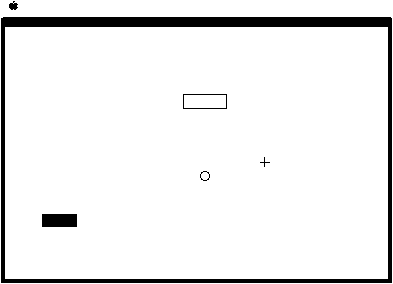Figure 4. Sample experimental condition.

If a move started before the solid bar stabilized, a beep was heard and the subject restarted the move. Subjects were instructed to balance speed and accuracy for an error rate around 4%. An examiner was present for all trials.

### Design

A fully within-subjects repeated measures design was used. Controlled variables were approach angle (THETA-A = 0°, 45°, & 90°), target amplitude (A = 2, 4, 8, 16, & 32 units), target width (W = 1, 2, 4, & 8 units), and target height (H = 1, 2, 4, & 8 units). Each unit mapped into 10 pixels for a maximum amplitude of 320 pixels (15.3 cm). Dependent variables were movement time (MT) and error rate (calculated from the x and y selection coordinates).

Only 78 of 240 possible cells were used. This kept the experiment manageable and exhausted a wide and important range of conditions. Twenty-six amplitude/size conditions (see Figure 5) were crossed with the three approach angles. Conditions with W = H (viz., squares) were excluded since they yield only small differences in IDs among the models tested. Amplitudes were selected in power-of-four increments starting at the larger of W or H. The latter requirement ensured that the starting position was outside the target for all approach angles.

```===========================================

Amplitudea

-------------------

Width   Height  2   4   8   16  32   No.

-------------------------------------------

2     1      *   .   *   .   *     3
4     1      .   *   .   *   .     2
8     1      .   .   *   .   *     2
1     2      *   .   *   .   *     3
4     2      .   *   .   *   .     2
8     2      .   .   *   .   *     2
1     4      .   *   .   *   .     2
2     4      .   *   .   *   .     2
8     4      .   .   *   .   *     2
1     8      .   .   *   .   *     2
2     8      .   .   *   .   *     2
4     8      .   .   *   .   *     2

--------

Total     26

============================================

a * = condition used; . = condition not used

```
Figure 5. Amplitude/size conditions used in the experiment

The 78 conditions were presented in random order until all were exhausted. This constituted one block. Fifteen blocks were administered over four days (3 + 4 + 4 + 4) for a total of 1170 trials per subject.

## RESULTS

A Newman-Keuls test using movement time and error rate as criterion variables showed no significant differences in the 15 block means. The data were then entered in a test for outliers, whereby trials with selection coordinates more than three standard deviations from the mean were eliminated. The deviations from the mean were expressed as 2D vectors using z = (x 2 + y 2)1 / 2. Of 14,040 total trials, 42 (0.3%) qualified as outliers and were removed.

### Approach Angle

Approach angle was the only factor fully crossed with other factors. Therefore, an analysis of variance was applied only to the main effect of approach angle on the dependent measures of movement time and error rate.

Trials were timed from the cursor leaving the start circle to the button-down action at the target. The grand mean for movement time was 743 ms. Moves along the horizontal and vertical axes were about the same (733 & 732 ms) while moves along the diagonal axis took 4% longer (MT = 764 ms, F2,22 = 23.86, p < .001).

Error rates were very close the optimal rating of 4%. The grand mean was 4.6%, with means along the horizontal, diagonal, and vertical axes of 3.9%, 5.1%, and 4.7% respectively. Statistical significance was achieved (F2,22 = 4.33, p < .05).

The above results were expected based on previous findings [e.g., 2]. Although the differences should be noted, they do not give one model an advantage since a range of short-and-wide and tall-and-narrow targets were used.

### Fit of the Models

Our main objective was to compare several interpretations of target width when the approach angle varies. Five models were tested:

```     Model           Target Width

------------------------------------------

STATUS QUO      horizontal extent (W)
W+H            sum of width and height
WxH            area
SMALLER-OF      smaller of width or height
W'             width  along line of approach

```
Each model was entered in a test of correlation and linear regression using the Shannon formulation for index of difficulty. The results are given in Figure 6.
```================================================================================

Regression  Coefficients

ID Range (bits)         -----------------------------------------

Model for     ----------------          SEb  Intercept,   Slope, b     IP
Target Width    Low    High      ra    (ms)   a (ms)       (ms/bit)     (bits/s)
--------------------------------------------------------------------------------
SMALLER-OF      1.58   5.04    .9501    64    230          166          6.0
W '             1.00   5.04    .9333    74    337          160          6.3
W+H             0.74   3.54    .8755    99    402          218          4.6
WxH             0.32   4.09    .8446    110   481          173          5.8
STATUS QUO      1.00   5.04    .8097    121   409          135          7.4
================================================================================
a n = 78, p < .001
b standard error of estimate```
Figure 6. Correlations and regression coefficients for five models for target width.
```==============================================================================

Comparison of Models for           Correlations of ID             Hotelling
Target Width                   with Movement Time             t testa
------------------------  ----------------------------------    ------------
1st Model    2nd Model    1st Model   2nd Model  Inter-Model     t     p
------------------------------------------------------------------------------
SMALLER-OF   W'            .9501      .9333      .8502           1.32  -
SMALLER-OF   STATUS QUO    .9501      .8097      .7881           6.31  .001
W'           STAUTS QUO    .9333      .8097      .6992           4.86  .001
==============================================================================
a two-tailed test, n = 78, df = 75```
Figure 7. Test for significant differences between models.

The correlations were above .8000 (p < .001) in all cases. The SMALLER-OF model had the highest correlation and the lowest standard error, while the STATUS QUO model (W) had the lowest r and the highest SE. Correlations and SEs for the W' model were comparable to those for the SMALLER-OF model. Performance indices (IP) were in the range of 4.6 to 7.4 bits/s. The intercepts were all positive with the SMALLER-OF model yielding the intercept closest to the origin.

The highest correlation in Figure 6 was for the SMALLER-OF model. Using this model we conclude that the predicted time (ms) to point to and select a rectangular target, regardless of approach angle, is

`    MT = 230 + 166 log2(A/W + 1),    (4)`
where W is the smaller of the target's width or height. Furthermore, the standard error of estimate can provide a 95% confidence window on the prediction. For example, a task rated at 5 bits of difficulty should take 230 + 166(5) +/- 2(64) ms; that is, between 932 ms and 1188 ms.

### Model Comparisons

Due to the ranking of correlations in Figure 6, further comparisons between the models were undertaken using Hotelling's t test for the difference between correlation coefficients [e.g., 7, p. 164]. The correlations were not significantly different between the STATUS QUO and W+H models (t = 2.00, df = 75, n.s.), or between the STATUS QUO and WxH models (t = 0.91, df = 75, n.s.). Therefore, the W+H and WxH models were excluded from further pair-wise comparisons.

The correlations for the STATUS QUO, SMALLER-OF, and W' models are compared in Figure 7. As evident, the correlation was significantly higher for the SMALLER-OF and W' models than for the STATUS QUO model (p < .001). Furthermore, the SMALLER-OF and W' models did not differ significantly from each other (p > .05). An initial conclusion, therefore, is that the SMALLER-OF and W' models are empirically superior to the STATUS QUO model. As noted earlier, the W' model is theoretically attractive since it retains the one-dimensionality of the model. In a practical sense, the SMALLER-OF model is appealing because it can be applied without consideration of approach angle. This is also true of the STATUS QUO model, but not of the W' model.

## DISCUSSION

Since these results are potentially important to researchers interested in applying Fitts' law to two-dimensional target acquisition tasks, discussions should continue in more detail. The role of target height and approach angle varies in each model and therefore the comparisons may not be equitable. For example, the STATUS QUO model does not use H and THETA-A. Is this a strength or a weakness in the model? In one sense, it is a strength, because fewer parameters brings generality and ease in application. On the other hand, if an additional and commonly varied parameter is shown to effect the dependent variable of interest, and the effect is to degrade a model's performance in comparison to another, then the absence of the extra parameter is a weakness. Of course, the conditions tested must be representative of the application. The present experiment measured the time to acquire rectangular targets in two-dimensional tasks. The levels of factors were not unlike those in interactive computer graphics systems, with the possible exception of text selection, where the majority of targets are short-and-wide (see below).

On generality, the same argument applies in comparing the SMALLER-OF and the W' models. Although applying the W' model requires A, W, H, and THETA-A, the SMALLER-OF model only considers A, W, and H. This is both a strength and a potential weakness in the SMALLER-OF model. Perhaps angles between 0° and 45°, for example, would yield variations in movement time more consistent with the W' model than the SMALLER-OF model. This remains to be tested. Nevertheless, the simplicity in applying the SMALLER-OF model with one less parameter is noteworthy.

In the limited case of text selection, targets are letters, words, or word sequences. The ubiquity of such tasks necessitates a model comparison under the relevant conditions; that is, with target height held constant.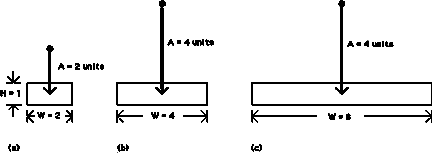By a STATUS QUO model, ID is a function only of A and W, where W is the width of the text object. So, as the number of characters increases, ID decreases (see Equations 1, 2, & 3) and, therefore, the predicted MT decreases. Is this a reasonable expectation? Intuitively, yes. After all, the larger a word, the easier it is to select! Upon closer examination however, it is evident that expectations disagree with predictions.

Considering only the STATUS QUO model initially, it is easy to demonstrate that erroneous predictions follow under extreme, yet reasonable, conditions. As noted earlier, a negative ID emerges using the Fitts or Welford formulation when A:W is less than 1:2. Although improbable for 1D tasks, this situation is perfectly common for 2D tasks. Using the Shannon formulation the limit in ID as W --> INFINITY is 0 bits. This seems reasonable, but as we now demonstrate, the rating for ID, although not negative, can become unrealistically low in 2D tasks using a STATUS QUO interpretation of target width.

Figure 8 is a clear refutation of applying the status quo definition -- that width = horizontal extent. Intuitively, target selection time for task (a) will be somewhat less than for task (b). But this is not predicted by a STATUS QUO model. Intuitively, target selection time for task (b) should be about the same as for task (c). Again, this is not predicted by a STATUS QUO model. Predictions do match expectations if the task difficulties are computed using the SMALLER-OF or W' model.

For further evidence, we need only examine the observations of Gillan et al. , who used conditions of W = 0.25, 1.0, 3.5, and 6.0 cm with H held constant at 0.5 cm (the height of a character). The targets were words or phrases of length 1, 5, 14, or 26 characters. The contour lines in Figure 9 support the SMALLER-OF model over the STATUS QUO model. The observed selection time decreased from the 1-character to the 5-character conditions for each amplitude condition (as expected for both models); however, MT remained the same across the 5-, 14-, and 26-character conditions. The latter effect, although not accounted for by the STATUS QUO model, is fully expected with the SMALLER-OF model because the target height was unchanging and consistently smaller than the target width. Gillan et al.'s  data clearly show that MT depends on A but not on W over the latter three conditions.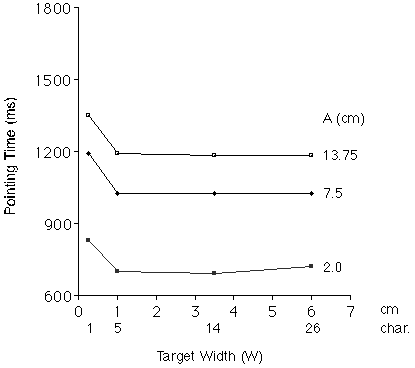Figure 9. Results from Gillan et al. . Movement time for the larger
three targets (where H < W) depends only on pointing distance,
as consistent with the SMALLER-OF model.

### The W' Model

The W' model, although slightly more difficult to apply, performed as well as the SMALLER-OF model in Figure 7. The model assumes that subjects move toward the centre of the target. No doubt, behavioural optimization would follow under extreme conditions, such as selecting a "very wide" target at close range. If the starting point is below at 45°, for example, movement distances could be reduced by advancing along a more direct path. Such extremes were not tested. To prevent biasing the comparisons for any one model, the experiment fully crossed the three approach angles with all A-W-H conditions; thus, the minimum amplitude for each condition could be applied at each approach angle. Conditions such as A = 2.0 cm and W = 6 cm (as used by Gillan et al., ) preclude the possibility of THETA-A = 0° since the starting position would be inside the target (see Figure 1). In fact, the designs employed by Card et al.  and Gillan et al.  would have provided even stronger evidence for the W' and SMALLER-OF models had their data been analyzed as in Figure 6 and Figure 7. The present design, whereby both short-and-wide and tall-and-narrow targets were fully crossed with 0°, 45°, and 90° approach angles, is unbiased with respect to the models tested.

Optimization trends were investigated by calculating the actual amplitudes and approach angles for all 78 conditions. As expected, optimization was most evident for the extreme short-and-wide and tall-and-narrow targets. The largest deviation occurred under the condition W = 1, H = 8, A = 8, and THETA-A = 45°, where means for the actual amplitude and approach angle were 7.1 units and 36.9°. For the vast majority of conditions, however, actual amplitudes and angles were remarkably close to the specified conditions. Analyses using actual measures for A and THETA-A were not pursued further.

When non-rectangular targets are used, applying the SMALLER-OF model is problematic; whereas, the W' model is applied in the usual way. Nevertheless, one can imagine odd-shaped targets without an obvious "centre". The W' model may yield unreasonably large or small estimates for target width in some instances. The area model (W x H) has some intuitive appeal in this case. Perhaps an odd-shaped target should be reduced to a minimum-circumference shape -- a circle -- having the same area. The WxH model would substitute the area for W, while the W' model would substitute the diameter.

## CONCLUSIONS

The results in Figure 6 and Figure 7 illustrate a problem with traditional applications of Fitts' law to two-dimensional target acquisition tasks. We have shown that the interpretation of target width and the formulation used in the calculation of a task's index of difficulty play a critical role in the accuracy of the model.

The Fitts and Welford formulations suffer by yielding a negative rating for a task's index of difficulty, particularly in 2D tasks since the A:W ratio can be very small. The Shannon formulation alleviates this by always providing a positive (or zero) rating for ID.

Consistently using the horizontal extent of a target as its "width" (the STATUS QUO view) also weakens the model and leads to inaccurate and sometimes erroneous predictions. Two models performed significantly better than the STATUS QUO model. The first -- the W' model -- substitutes for W the extent of the target along an approach vector through the centre. This model is theoretically attractive since it retains the one-dimensionality of Fitts' law; however, the approach angle (as well as the width, height, and amplitude) must be known a priori. The second -- the SMALLER-OF model -- substitutes for W either the width or height of the target, whichever is smaller. This model is easy to apply, but is limited to rectangular targets, unlike the W' model. Both models, in tests of correlation, performed significantly better than the STATUS QUO model; however, no difference was detected between them. These findings should prove useful in subsequent applications of Fitts' law to target acquisitions tasks on computing systems with graphical user interfaces.

## ACKNOWLEDGEMENT

This research was supported by the Natural Sciences and Engineering Research Council of Canada, Xerox Palo Alto Research Center, Digital Equipment Corp., and Apple Computer Inc. We gratefully acknowledge this contribution, without which, this work would not have been possible.

## REFERENCES

1. Boritz, J., Booth, K. S., & Cowan, W. B. (1991). Fitts's law studies of directional mouse movement. Proceedings of Graphics Interface `91, 216-223. Toronto: CIPS.

2. Card, S. K., English, W. K., & Burr, B. J. (1978). Evaluation of mouse, rate-controlled isometric joystick, step keys, and text keys for text selection on a CRT. Ergonomics, 21, 601-613.

3. Epps, B. W. (1986). Comparison of six cursor control devices based on Fitts' law models. Proceedings of the 30th Annual Meeting of the Human Factors Society, 327-331.

4. Fitts, P. M. (1954). The information capacity of the human motor system in controlling the amplitude of movement. Journal of Experimental Psychology, 47, 381-391.

5. Fitts, P. M., & Peterson, J. R. (1964). Information capacity of discrete motor responses. Journal of Experimental Psychology, 67, 103-112.

6. Gillan, D. J., Holden, K., Adam, S., Rudisill, M., & Magee, L. (1990). How does Fitts' law fit pointing and dragging? Proceedings of the CHI '90 Conference on Human Factors in Computing Systems, 227-234. New York: ACM.

7. Guilford, J. P., & Fruchter, B. (1978). Fundamental statistics in psychology and education (6th ed.). New York: McGraw-Hill.

8.Jagacinski, R. J., & Monk, D. L. (1985). Fitts' law in two dimensions with hand and head movements. Journal of Motor Behavior, 17, 77-95.

9. Jellinek, H. D., & Card, S. K. (1990). Powermice and user performance. Proceedings of the CHI '90 Conference on Human Factors in Computing Systems, 213-220. New York: ACM.

10. MacKenzie, I. S. (in press). Fitts' law as a research and design tool in human-computer interaction. Human-Computer Interaction.

11.MacKenzie, I. S. (1989). A note on the information-theoretic basis for Fitts' law. Journal of Motor Behavior, 21, 323-330.

12. MacKenzie, I. S., Sellen, A., & Buxton, W. (1991). A comparison of input devices in elemental pointing and dragging tasks. Proceedings of the CHI '91 Conference on Human Factors in Computing Systems, 161-166. New York: ACM.

13. Sheridan, T. B., & Ferrell, W. R. (1963). Remote manipulative control with transmission delay. IEEE Transactions on Human Factors in Electronics, 4, 25-29.

14. Ware, C., & Mikaelian, H. H. (1989). A evaluation of an eye tracker as a device for computer input. Proceedings of the CHI+GI '87 Conference on Human Factors in Computing Systems and Graphics Interface, 183-188. New York: ACM.

15. Welford, A. T. (1968). Fundamentals of skill. London: Methuen.